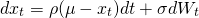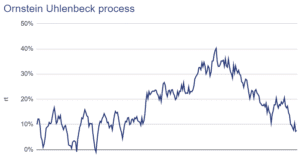# Ornstein Uhlenbeck process

The Ornstein-Uhlenbeck process is a stochastic process that exhibits mean-reverting behaviour. In particular, the Ornstein-Uhlenbeck model forces the process to revert to its long-term mean. It is often used to value derivatives for which no closed-form solution exists. The main advantage of the Ornstein-Uhlenbeck process is that is more closely resembles the behaviour of securities. The process was proposed by Leonard Ornstein and George Uhlenbeck.

On this page, we discuss the process, discuss the Ornstein-Uhlenbeck formula and finally implement the model in Excel. The Excel spreadsheet is available for download at the bottom of the page.

## Ornstein Uhlenbeck process definition

The OU-process can be considered a modification of the traditional random walk or Wiener process that is commonly assumed for the pricing of derivatives. The process satisfies the following equationWhere rho > 0, sigma > 0, and Wt is simple a Wiener process. Sometimes, the above definition is also referred to as the Vasicek model. For those familiar with econometrics, the process is the continuous-time equivalent of an AR(1) process.

## Ornstein-Uhlenbeck applications

While the process was first described in the context of physics, it is also used very often by quants in the context of finance. The model can be used to model securities or rates that exhibit mean-reverting behaviour. This is useful for example to model interest rates, commodity prices, currencies, and pairs trading. A famous example of a model that uses an Ornstein-Uhlenbeck process is the Vasicek model, which models interest rates. In this model as well as the other models, the mean mu is the expected return, sigma is the volatility caused by new arriving, and rho is the rate at which the shocks disappear again.

The following figure illustrates a number of OU processes. The spreadsheet is available for download at the bottom of the page.## Ornstein-Uhlenbeck in Excel

We can implement the model in discrete time in Excel. The Excel spreadsheet illustrates how to generate time series of a security using an Ornstein-Uhlenbeck stochastic process. The resulting price paths can then be used to simulate the returns of an underlying. The price of the underlying can then be used for derivative pricing.

## Summary

We discussed the OU process, a variation to the traditional random walk. The advantage of the OU process is that it exhibits mean-reversion, an important characteristic of a number of financial markets. The process is used a lot in finance and can even be considered the dominant model in many fields in finance.